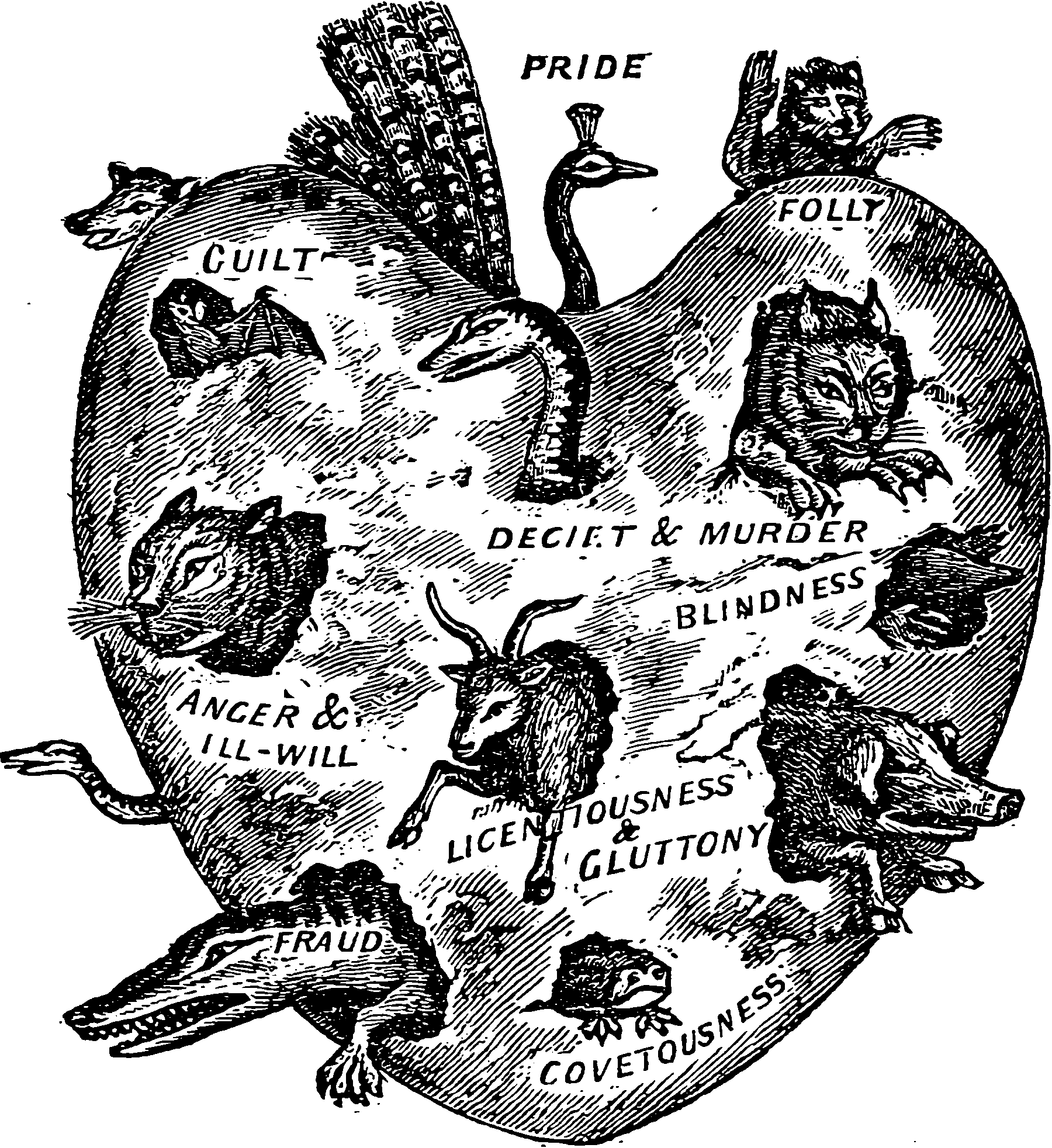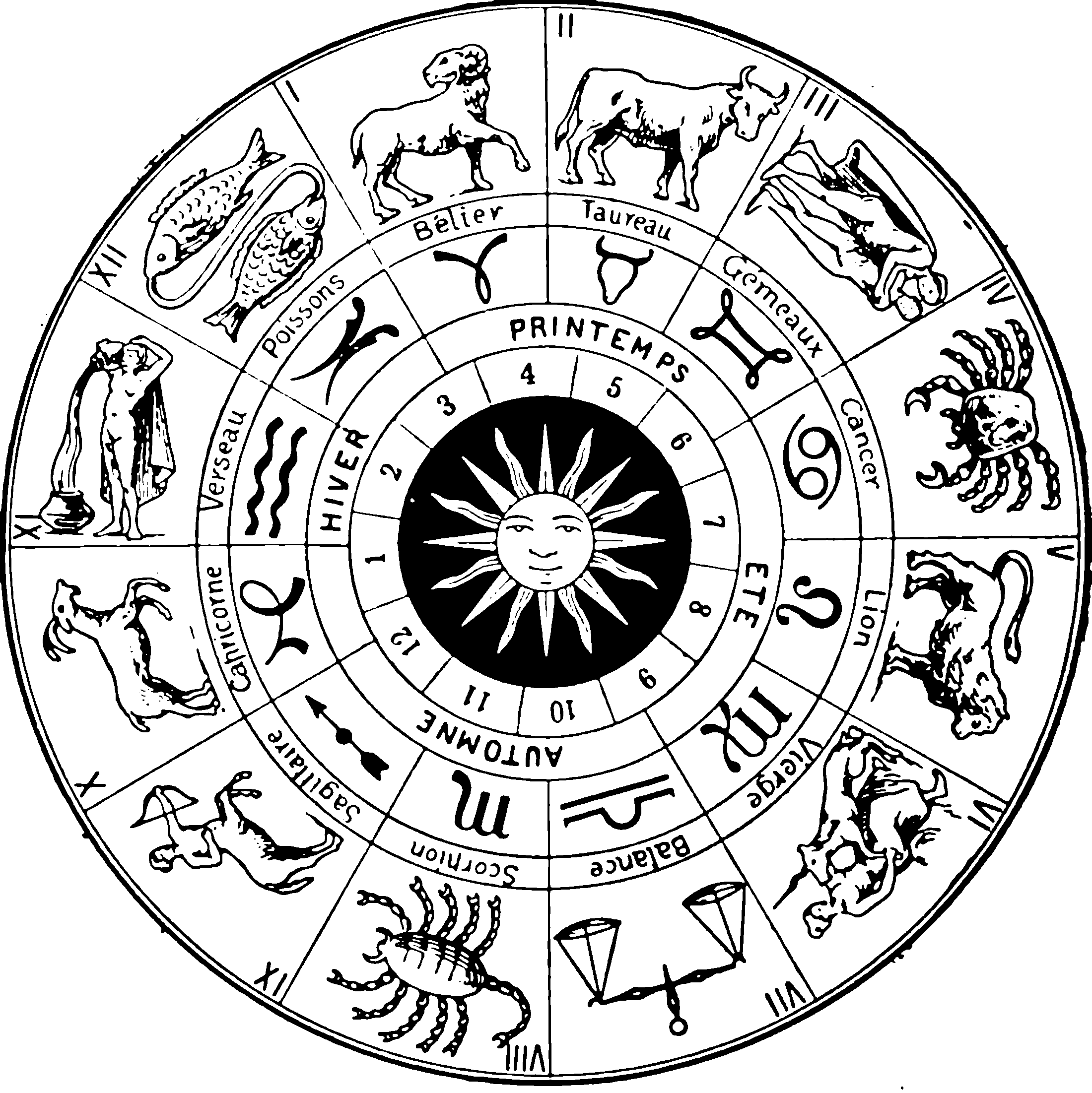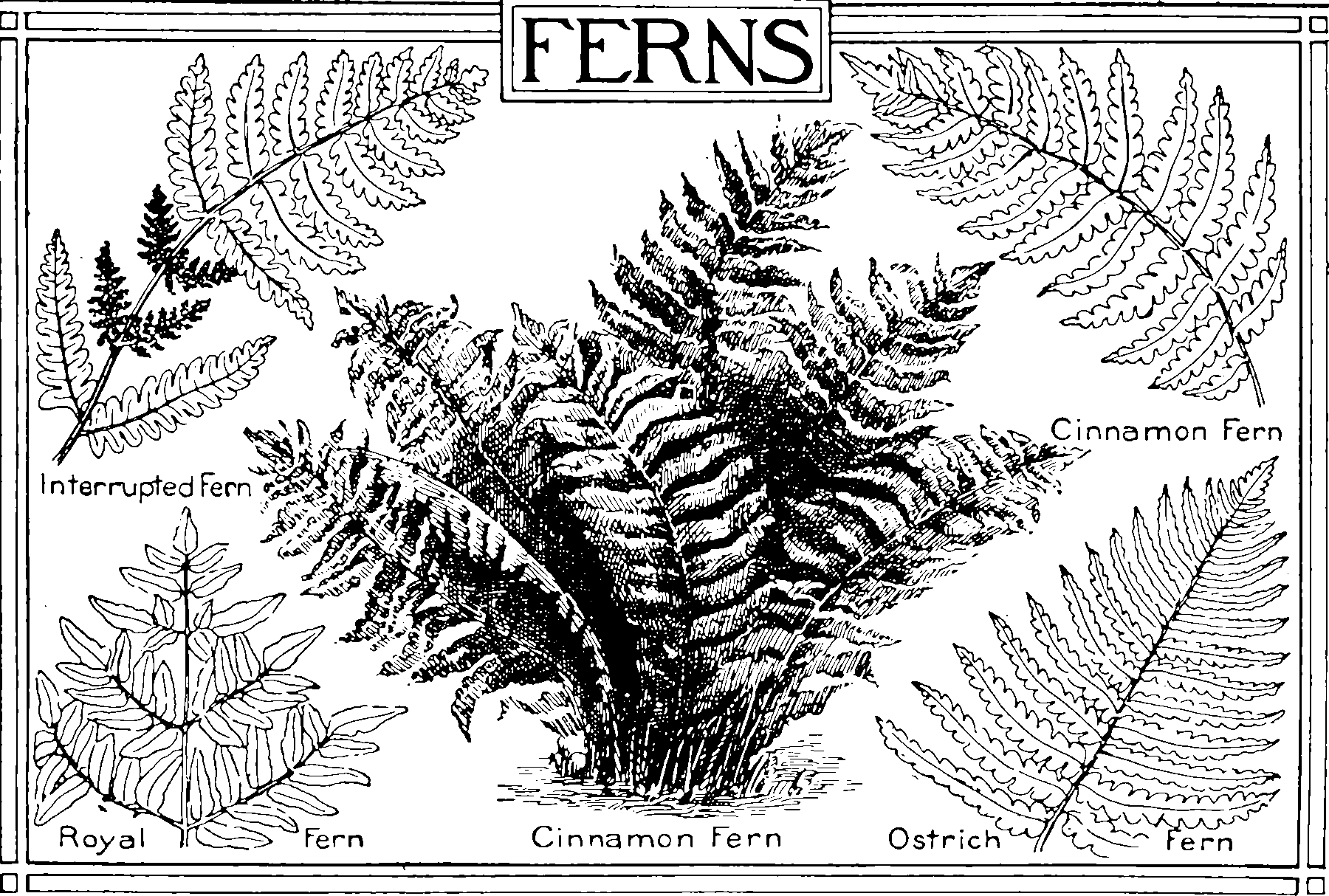# Categorical random variatesDistributions over categories.

## Stick breaking tricks

Recommended further reading: Machine Learning Trick of the Day (6): Tricks with Sticks— Shakir Mohammed.

## via random measures

See random measures.

See Pólya-Gamma.

## Multicategorical distributions

Can something belong to many categories? Then we are probably looking for Paintbox models or some kind of multivariate Bernoulli model.

## OrdinalIf there is a natural ordering to the categories, then we are in a weird place.

## Calibration

Kenneth Tay says

In the context of binary classification, calibration refers to the process of transforming the output scores from a binary classifier to class probabilities. If we think of the classifier as a “black box” that transforms input data into a score, we can think of calibration as a post-processing step that converts the score into a probability of the observation belonging to class 1.

The scores from some classifiers can already be interpreted as probabilities (e.g. logistic regression), while the scores from some classifiers require an additional calibration step before they can be interpreted as such (e.g. support vector machines).

He recommends the tutorial Huang et al. (2020) and associated github.

## Dirichlet distribution

TBD. See Dirichlet distributions.

## Dirichlet process

TBD. A distribution over an unknown number of categories. See also Gamma processes, which is how I learned to understand Dirichlet processes, insofar as I do.

## Parametric distributions over non-negative integers

See count models.

## Hierarchical

TBD### No comments yet. Why not leave one?

GitHub-flavored Markdown & a sane subset of HTML is supported.